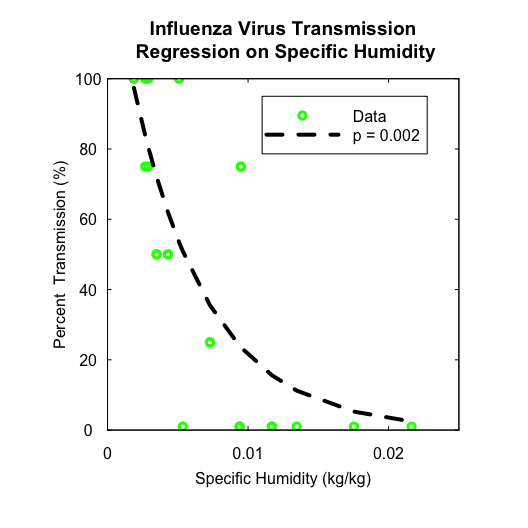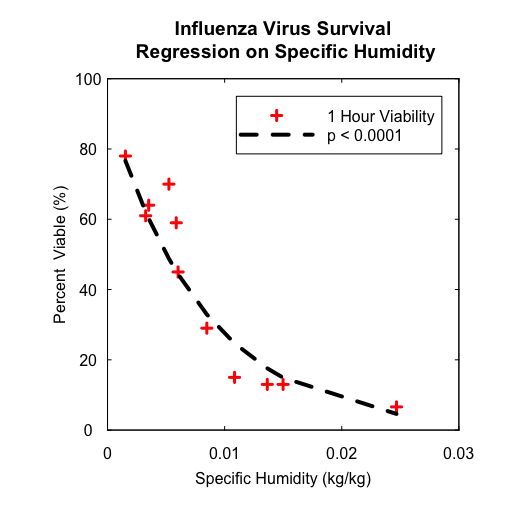# Regressions of Influenza A Virus Aerosol Transmission and Survival on Specific Humidity

#### 2019-11-10

Through reanalysing laboratory data from previous studies (Harper 1961; Lowen et al. 2007, 2008), Shaman and Kohn (2009) found that regression models using absolute humidity (AH) could explain more variability in both influenza virus transmission (IVT) and influenza virus survival (IVS) than those models using relative humidity (RH). This vignette demonstrates the functionality of humidity pacakge by reproducing their log-linear regressions of IVT and IVS data on specific humidity (SH).

## Regression of IVT on SH

Using the guinea pig model, (Lowen et al. 2007, 2008) directly tested the hypothesis that temperature and RH impact the influenza virus transmission efficiency by performing 24 transmissionn experiments at RH from 20% to 80% and 5°C, 20°C, or 30°C. The data from transmission experiments indicated that both cold nand dry conditions favor the aerosol transmission of influenza virus. These data are collated from the two papers and now are stored as package data of humidity with the name of ivt. In the following, the dataset ivt is used to explore the relationship between IVT and SH. As SH is not provided, we firstly apply functions from humidity package to calculate SH from temperature and RH.

# load humidity package
library(humidity)

# display built-in guinea pig airborne influenza virus transmission data
str(ivt)
## 'data.frame':    24 obs. of  4 variables:
##  $T : num 20 20 20 20 20 20 20 20 20 20 ... ##$ RH    : num  20 20 35 35 50 50 65 65 80 80 ...
##  $PT : num 100 75 100 100 25 25 75 75 0 0 ... ##$ source: chr  "Lowen.etal-2007" "Lowen.etal-2007" "Lowen.etal-2007" "Lowen.etal-2007" ...

As the temperature T is in degree Celsius (°C), we first apply C2K function to convert the temperature into Kelvin (K) before our following calculation. Then we call SVP and WVP functions to calculate saturation vapor pressure $$e_s$$ (hPa) and partial water vapor pressure $$e$$ (Pa) at temperature $$T$$, respectively. Finally by calling AH, SH, and MR functions, we can calculate humidity measures of interest, such as AH $$\rho_w$$ ($$\mathrm{kg/m^3}$$), SH $$q$$ (kg/kg), and mixing ratio $$\omega$$ (kg/kg).

Note that SVP function provides two formulas (either Clausius-Clapeyron equation or Murray equation) for calculating saturation vapor pressure. Both results are the same and the default formula is “Clausius-Clapeyron”, which is consistent with Shaman and Kohn (2009). Furthermore, because (Lowen et al. 2007, 2008) didn’t give any information on the atmospheric pressure condition under which their transmission experiments were conducted, we just assume that they performed these experiments under standard atmospheric pressure. Thus, the default value of p = 101325 Pa is used in SH function when calculating specific humidity.

It is noted that no aerosol transmission of influenza virus was observed at 30°C and all RHs. The transmission values of 0 result in the fitting failure of log-linear regression. Thus, a small quantity of 1 is added to them to avoid taking log of 0.

library(dplyr)
ivt <- ivt %>%
mutate(Tk = C2K(T), # tempature in Kelvin
Es = SVP(Tk), # saturation vapor pressure in hPa
E = WVP2(RH, Es), # partial water vapor pressure in Pa
rho = AH(E, Tk), # absolute humidity in kg/m^3
q = SH(E), # specific humidity in kg/kg
omega = MR(q), # mixing ratio in kg/kg
) %>%
mutate(PT = ifelse(PT == 0, 1, PT)) # add a small quantity to avoid taking log of zero
# check the calculation results
head(ivt)
##    T RH  PT          source     Tk       Es         E         rho
## 1 20 20 100 Lowen.etal-2007 293.15 23.63899  472.7798 0.003494447
## 2 20 20  75 Lowen.etal-2007 293.15 23.63899  472.7798 0.003494447
## 3 20 35 100 Lowen.etal-2007 293.15 23.63899  827.3646 0.006115282
## 4 20 35 100 Lowen.etal-2007 293.15 23.63899  827.3646 0.006115282
## 5 20 50  25 Lowen.etal-2007 293.15 23.63899 1181.9494 0.008736118
## 6 20 50  25 Lowen.etal-2007 293.15 23.63899 1181.9494 0.008736118
##             q       omega
## 1 0.002907371 0.002915848
## 2 0.002907371 0.002915848
## 3 0.005094650 0.005120738
## 4 0.005094650 0.005120738
## 5 0.007287741 0.007341242
## 6 0.007287741 0.007341242

Moreover, those zero transmission efficiencies have a significant influence on the coefficient estimate of SH. Therefore, instead of a linear regression model with the log transformed response variable, a GLM model with Gaussian family and log link is used to obtain a robust coefficient estimate.

# log-linear regression of influenza virus airborne  transmission on specific humididty
loglm <- glm(PT ~ q, family = gaussian(link = "log"), data = ivt)
summary(loglm)
##
## Call:
## glm(formula = PT ~ q, family = gaussian(link = "log"), data = ivt)
##
## Deviance Residuals:
##     Min       1Q   Median       3Q      Max
## -49.906  -14.616   -9.527    5.985   51.440
##
## Coefficients:
##              Estimate Std. Error t value Pr(>|t|)
## (Intercept)    4.9291     0.2077  23.733  < 2e-16 ***
## q           -186.5339    52.7765  -3.534  0.00186 **
## ---
## Signif. codes:  0 '***' 0.001 '**' 0.01 '*' 0.05 '.' 0.1 ' ' 1
##
## (Dispersion parameter for gaussian family taken to be 726.2067)
##
##     Null deviance: 37884  on 23  degrees of freedom
## Residual deviance: 15977  on 22  degrees of freedom
## AIC: 230.13
##
## Number of Fisher Scoring iterations: 6

The regression coefficient of SH $$q$$ is significant with a $$p$$-value equal to 0.0019, which is the same as that annotated in Fig. 1 (F) of Shaman and Kohn (2009). Furthermore, the coefficient estimate for SH $$q$$ is -187, which is very close to the value of $$a = -180$$ that was finally used in the functional relationship between $$R_0 (t)$$ and $$q(t)$$ per Equation 4 in Shaman et al. (2010).

The following codes plot the aerosol transmission efficiency as a function of SH with the log-linear regression curve overlapped.

# get fitted value to plot regression curve
ivt$fit.val <- exp(predict(loglm)) ivt <- ivt[with(ivt, order(q)), ] # plot percentage viable virus vs. specific humidity par(pty = "s") plot(x = ivt$q, y = ivt$PT, col = "green", pch = 1, lwd = 3, xaxt = "n", yaxt = "n", xlim = c(0, 0.025), ylim = c(0, 100), xaxs = "i", yaxs = "i", xlab = "", ylab = "", main = "Influenza Virus Transmission\n Regression on Specific Humidity") title(xlab = "Specific Humidity (kg/kg)", ylab = "Percent Transmission (%)", mgp = c(2, 1, 0)) # plot regression curve lines(x = ivt$q, y = ivt$fit.val, lty = "dashed", lwd = 4) axis(side = 1, at = seq(0, 0.03, by = 0.01), labels = c("0", "0.01", "0.02", "0.03"), tck = 0.01, padj = -0.5) axis(side = 2, at = seq(0, 100, by = 20), tck = 0.01, las = 2, hadj = 0.5) axis(side = 3, at = seq(0, 0.03, by = 0.01), labels = FALSE, tck = 0.01) axis(side = 4, at = seq(0, 100, by = 20), labels = FALSE, tck = 0.01) legend(0.011, 95, legend = c("Data", "p = 0.002"), pch = c(1, NA), col = c("green", "black"), lty = c(NA, "dashed"), lwd = c(3, 4), seg.len = 5)The above figure is also the reproduction of Figure 1.(A) of Shaman et al. (2010). ## Regression of IVS on SH Harper (1961) tested airborne virus particles of influenza A for viable survival in the dark at controlled temperature and RH for up to 23 hour after spraying. The 1-h IVS data are extracted from the paper and now are stored as package data of humidity with the name of ivs. Using the dataset ivs, we explore the relationship between IVS and SH. Likewise, we first calculate various AH measures by calling corrresponding functions from humidity package. # display built-in 1-h influenza virus surival data str(ivs) ## 'data.frame': 11 obs. of 3 variables: ##$ T : num  7.5 7.5 7.5 22.2 22.2 ...
##  $RH: num 24 51 82 21 35 50.5 64.5 81 20 49.5 ... ##$ PV: num  78 61 70 64 59 29 15 13 45 13 ...
ivs <- ivs %>%
mutate(Tk = C2K(T), # tempature in Kelvin
Es = SVP(Tk), # saturation vapor pressure in hPa
E = WVP2(RH, Es), # partial water vapor pressure in Pa
rho = AH(E, Tk), # absolute humidity in kg/m^3
q = SH(E), # specific humidity in kg/kg
omega = MR(q), # mixing ratio in kg/kg
)
# check the calculation results
head(ivs)
##       T   RH PV     Tk       Es         E         rho           q
## 1  7.50 24.0 78 280.65 10.38008  249.1219 0.001923341 0.001530702
## 2  7.50 51.0 61 280.65 10.38008  529.3840 0.004087100 0.003256149
## 3  7.50 82.0 70 280.65 10.38008  851.1665 0.006571416 0.005241681
## 4 22.25 21.0 64 295.40 27.21156  571.4428 0.004191522 0.003515398
## 5 22.25 35.0 59 295.40 27.21156  952.4047 0.006985870 0.005867353
## 6 22.25 50.5 29 295.40 27.21156 1374.1839 0.010079613 0.008479141
##         omega
## 1 0.001533048
## 2 0.003266786
## 3 0.005269301
## 4 0.003527799
## 5 0.005901982
## 6 0.008551651

As the percentages of viable virus are all $$\gt 0$$, the coefficient estimates using a linear model with log-transformed response variable are close to those estimated from a GLM model with Gaussian family and log link (results not shown). Herein, we present the results from the former model.

# log-linear regression of 1-h infuenza A virus survival on specific humididty
loglm <- lm(log(PV) ~ q, data = ivs)
summary(loglm)
##
## Call:
## lm(formula = log(PV) ~ q, data = ivs)
##
## Residuals:
##      Min       1Q   Median       3Q      Max
## -0.49834 -0.13209  0.01414  0.16364  0.36192
##
## Coefficients:
##              Estimate Std. Error t value Pr(>|t|)
## (Intercept)    4.5249     0.1445  31.323 1.69e-10 ***
## q           -121.5782    13.1247  -9.263 6.74e-06 ***
## ---
## Signif. codes:  0 '***' 0.001 '**' 0.01 '*' 0.05 '.' 0.1 ' ' 1
##
## Residual standard error: 0.281 on 9 degrees of freedom
## Multiple R-squared:  0.9051, Adjusted R-squared:  0.8945
## F-statistic: 85.81 on 1 and 9 DF,  p-value: 6.74e-06

The regression coefficient of SH $$q$$ is significant with $$p < 0.0001$$ being the same as the $$p$$-value indicated in Figure 1.(B) of Shaman et al. (2010).

The following codes draw the scatter plot of 1-h IVS vs. SH with the log-linear regression curve overlapped, which also reproduce Figure 1.(B) of Shaman et al. (2010).

# get fitted value to plot regression curve
ivs$fit.val <- exp(predict(loglm)) ivs <- ivs[with(ivs, order(q)), ] # plot percentage viable virus vs. specific humidity par(pty = "s") plot(x = ivs$q, y = ivs$PV, col = "red", pch = 3, lwd = 3, xaxt = "n", yaxt = "n", xlim = c(0, 0.03), ylim = c(0, 100), xaxs = "i", yaxs = "i", xlab = "", ylab = "", main = "Influenza Virus Survival\n Regression on Specific Humidity") title(xlab = "Specific Humidity (kg/kg)", ylab = "Percent Viable (%)", mgp = c(2, 1, 0)) # plot regression curve lines(x = ivs$q, y = ivs\$fit.val, lty = "dashed", lwd = 4)
axis(side = 1, at = seq(0, 0.03, by = 0.01), labels = c("0", "0.01", "0.02", "0.03"),
tck = 0.01, padj = -0.5)
axis(side = 2, at = seq(0, 100, by = 20), tck = 0.01, las = 2, hadj = 0.5)
axis(side = 3, at = seq(0, 0.03, by = 0.01), labels = FALSE, tck = 0.01)
axis(side = 4, at = seq(0, 100, by = 20), labels = FALSE, tck = 0.01)
legend(0.011, 95, legend = c("1 Hour Viability", "p < 0.0001"), pch = c(3, NA),
col = c("red", "black"), lty = c(NA, "dashed"), lwd = c(3, 4), seg.len = 5)As shown in the above two log-linear regressions of IVT and IVS data on SH, the almost equivalent coefficient estimates and the similar $$p$$-values verify the correctness of various AH measures calculated by humidity package.

The initial version of this vignette included only the log-linear regression of IVS on SH. Thank Dr. Andrei R. Akhmetzhanov from Hokkaido University, Japan for pointing out that the final choice of $$a = -180$$ in Shaman et al. (2010) was based on the log-linear regression of IVT on SH.